# Visualising Solid Shapes Class 8 Notes: Chapter 10

## Introduction

Visualising Solid Shapes Class 8 Notes provided here are the right tool for students to study productively and score better marks in the exams. Chapter 10 notes will help students get a complete overview of the chapter and also a clear insight into the important topics to remember. The CBSE notes further come with detailed information about each topic and students can have an effective revision before the exams.

### Two Dimensional Object

A shape with only two dimensions (such as length and width) and no thickness is called two-dimensional shape. Squares, Circles, Triangles, etc are two dimensional objects. Also known as “2D”.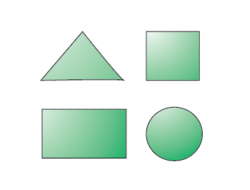To know more about 2D Shapes, visit here.

### Area

Area is measurement of space enclosed by a closed geometrical figure.

#### For More Information On Visualizing Solid Shapes and Area, Watch The Below Video.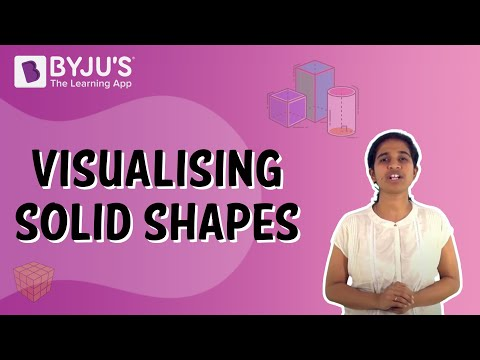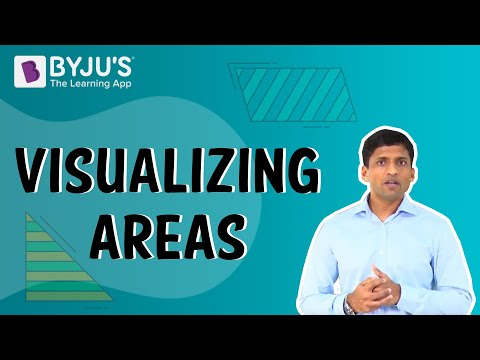To know more about Area of Different Shapes, visit here.

### Volume

Volume is measurement of total space occupied by a solid.

To know more about Volume Formulas, visit here.

### Three Dimensional Objects- Solids

Shapes which can be measured in 3 directions are called three-dimensional shapes. These shapes are also called solid shapes. Length, width, and height (or depth or thickness) are their dimensions.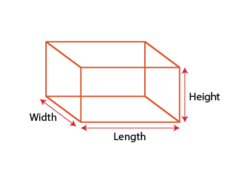To know more about 3D Shapes, visit here.

### Formation of Solid Solids

Stacking of 2 dimensional figures, results in solids shapes.
Example: Linear stacking of circles, forms the solid shape called cylinder.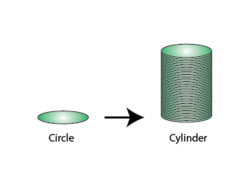## Solids and Their Classification

### Hexagon

In geometry, hexagon is a polygon with 6 sides. Sum of all interior angles is equal to 720°.
If all the sides of hexagon are equal then it is called regular hexagon, then each interior angle measures 120°.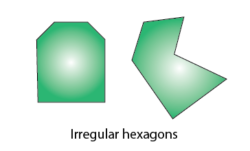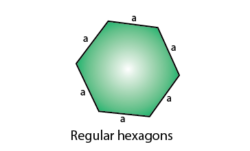### Non-Polyhedrons

Solids with curved faces are called Non polyhedrons. They also can be discribed as solids which have sides that are not polygons.
Example: Sphere, Cylinder, Cone, etc.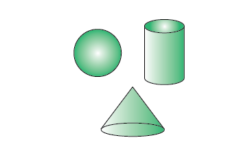### Polyhedrons

A Polyhedron is a solid in three dimensions with flat polygonal faces, straight edges and sharp corners or vertices. In short, Solids with flat surfaces are called Polyhedrons.(or Polyhedra)

Regular polyhedron: All faces constitute regular polygons and at each vertex the same number of faces intersect. Example : Cube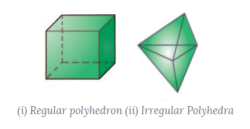### Solid Cuboid

A cuboid is a solid bounded by six rectangular plane regions.
It is formed by stacking rectangles linearly.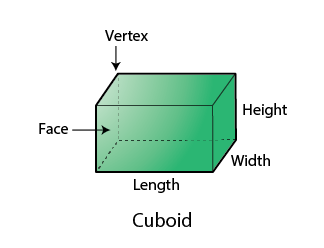#### For More Information On Cube and Cuboid, Watch The Below Video.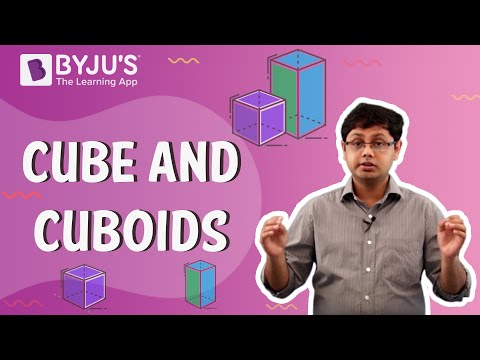### Solid Triangular Prism

Solid Triangular Prism: A polyhedron with two triangular  bases parallel to each other. It is formed by stacking triangles linearly.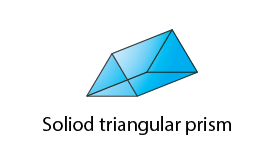### Solid Hexagonal Prism

Solid Hexagonal Prism: A polyhedron with two hexagonal  bases parallel to each other. It is formed by stacking hexagons linearly. Each face of hexagonal prism is rectangular in shape.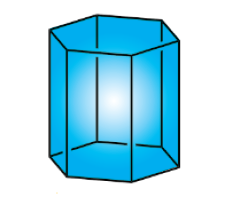### Solid Cylinder

Solid cylinder can be formed in two different ways:

(i) By stacking rectangles in a circular fashion.

(ii) By stacking many circles linearly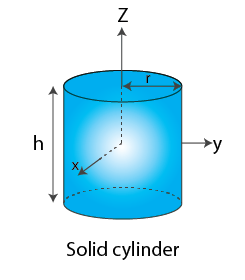### Solid Sphere

Solid spheres are formed by stacking circles in a circular fashion.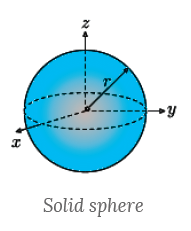### Solid Cone

Solid Cones are formed by stacking triangles which are right-angled, in a circular fashion with edge which is right angled at the center.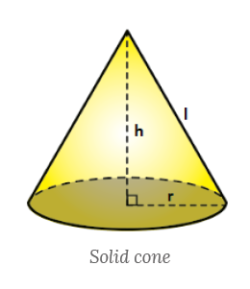### Formation of Hollow Solids

Hollow Solids are obtained by joining two dimensional figures.

### Hollow Cuboid

Hollow Cuboid: Formed by joining six rectangles in a specific manner as shown below: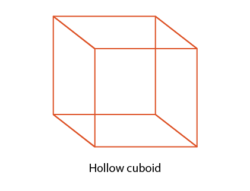### Hollow Triangular Prism

Hollow triangular prism: Formed by joining two triangles and three rectangles in a specific manner as shown below: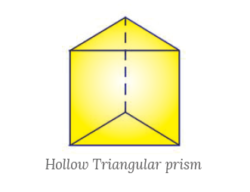### Hollow Hexagonal Prism

Hollow hexagonal Prism: Formed by joining two hexagons and six rectangles as shown below: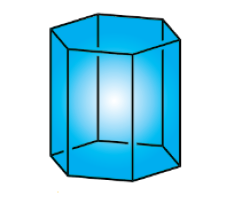Hollow Hexagonal Prism

### Hollow Cylinder

Hollow Cylinder: A cylinder is made by rotating a rectangle around either its length or breadth as shown below.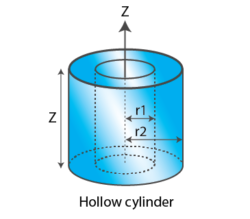### Hollow Cone

Hollow Cone: A circle and a curved sector of a circle are joined together as shown below: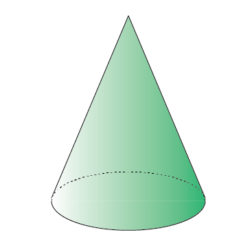Hollow Cone

### Pyramid

Pyramid: All side faces are triangular in shape and base is of the shape of any polygon.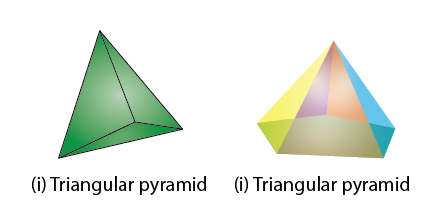### Types of Polyhedrons

Polyhedrons are of two types:

(i) Convex Polyhedron:
A polyhedron whose surface (comprising its faces, edges and vertices) does not intersect itself.
Line segment joining any two points of the polyhedron lies within its interior part or on surface.